Case Based Questions Test: Introduction to Trigonometry

# Case Based Questions Test: Introduction to Trigonometry

Test Description

## 10 Questions MCQ Test | Case Based Questions Test: Introduction to Trigonometry

Case Based Questions Test: Introduction to Trigonometry for Class 10 2023 is part of Class 10 preparation. The Case Based Questions Test: Introduction to Trigonometry questions and answers have been prepared according to the Class 10 exam syllabus.The Case Based Questions Test: Introduction to Trigonometry MCQs are made for Class 10 2023 Exam. Find important definitions, questions, notes, meanings, examples, exercises, MCQs and online tests for Case Based Questions Test: Introduction to Trigonometry below.
Solutions of Case Based Questions Test: Introduction to Trigonometry questions in English are available as part of our course for Class 10 & Case Based Questions Test: Introduction to Trigonometry solutions in Hindi for Class 10 course. Download more important topics, notes, lectures and mock test series for Class 10 Exam by signing up for free. Attempt Case Based Questions Test: Introduction to Trigonometry | 10 questions in 20 minutes | Mock test for Class 10 preparation | Free important questions MCQ to study for Class 10 Exam | Download free PDF with solutions
 1 Crore+ students have signed up on EduRev. Have you?
Case Based Questions Test: Introduction to Trigonometry - Question 1

### Read the following text and answer the following question on the basis of the same:Authority wants to construct a slide in a city park for children. The slide was to be constructed for children below the age of 12 years. Authority prefers the top of the slide at a height of 4 m above the ground and inclined at an angle of 30° to the ground.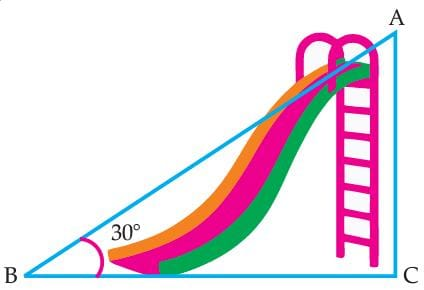Q. In the given figure, the value of (sin C × cos A) is:

Detailed Solution for Case Based Questions Test: Introduction to Trigonometry - Question 1 Since, AC ⊥ BC, then ∠C = 90°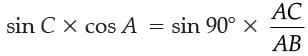= 1 x 4 / 8

= 1 / 2

Case Based Questions Test: Introduction to Trigonometry - Question 2

### Read the following text and answer the following question on the basis of the same:Authority wants to construct a slide in a city park for children. The slide was to be constructed for children below the age of 12 years. Authority prefers the top of the slide at a height of 4 m above the ground and inclined at an angle of 30° to the ground.Q. The distance of AB is :

Detailed Solution for Case Based Questions Test: Introduction to Trigonometry - Question 2 We have,

∠B = 30° and AC = 4 m

Then, sin 30° = AC / AB

⇒ 1 / 2 = 4 / AB

⇒ AB = 8 m.

Case Based Questions Test: Introduction to Trigonometry - Question 3

### Read the following text and answer the following question on the basis of the same:Authority wants to construct a slide in a city park for children. The slide was to be constructed for children below the age of 12 years. Authority prefers the top of the slide at a height of 4 m above the ground and inclined at an angle of 30° to the ground.Q. In the given figure, if AB + BC = 25 cm and AC = 5 cm, then the value of BC is:

Detailed Solution for Case Based Questions Test: Introduction to Trigonometry - Question 3 We have,

∠C = 90°

AB + BC = 25 cm and AC = 5 cm

Let BC be x cm, then AB = (25 – x) cm

By using Pythagoras theorem,

AB2 = BC2 + AC2

⇒ (25 – x2) = x2 + (5)2

⇒ 625 – 50x + x2 = x2 + 25

⇒ 50x = 600

⇒ x = 600 / 50 = 12

Hence, BC = 12 cm

Case Based Questions Test: Introduction to Trigonometry - Question 4

Read the following text and answer the following question on the basis of the same:

Authority wants to construct a slide in a city park for children. The slide was to be constructed for children below the age of 12 years. Authority prefers the top of the slide at a height of 4 m above the ground and inclined at an angle of 30° to the ground.Q. The value of sin2 30° + cos2 60° is:

Detailed Solution for Case Based Questions Test: Introduction to Trigonometry - Question 4 sin2 30° + cos2 60°

= (1 / 2)2 + (1 / 2)2

= 1 / 4 + 1 / 4

= 2 / 4 = 1 / 2

Case Based Questions Test: Introduction to Trigonometry - Question 5

Read the following text and answer the following question on the basis of the same:

Authority wants to construct a slide in a city park for children. The slide was to be constructed for children below the age of 12 years. Authority prefers the top of the slide at a height of 4 m above the ground and inclined at an angle of 30° to the ground.Q. If cos A = 1 / 2 , then the value of 12 cot2 A – 2 is:

Detailed Solution for Case Based Questions Test: Introduction to Trigonometry - Question 5 cos A = 1 / 2

⇒ cos A = cos 60°

⇒ A = 60°

Then 12 cot2 A – 2 = 12(cot 60°) – 2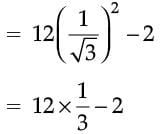= 4 – 2 = 2.

Case Based Questions Test: Introduction to Trigonometry - Question 6

Read the following text and answer the following question on the basis of the same:

'Skysails' is that genre of engineering science that uses extensive utilization of wind energy to move a vessel in the sea water. The sky sails technology allows the towing kite to gain a height of anything between 100 m to 300 m. The sailing kite is made in such a way that it can be raised to its proper elevation and then brought back with the help of a telescopic mast that enables the kite to be raised properly and effectively.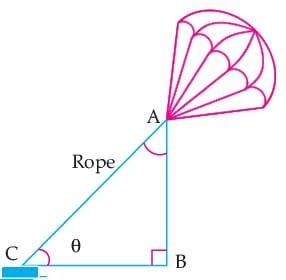Q. If cos A = 1/ 2 , then the value of 9 cot2 A – 1 is:

Detailed Solution for Case Based Questions Test: Introduction to Trigonometry - Question 6 Given, cos A = 1/ 2

⇒ cos A = cos 60°

⇒ A = 60°

Then, 9 cot2 A – 1 = 9(cot 60°)2 – 1= 2

Case Based Questions Test: Introduction to Trigonometry - Question 7

Read the following text and answer the following question on the basis of the same:

'Skysails' is that genre of engineering science that uses extensive utilization of wind energy to move a vessel in the sea water. The sky sails technology allows the towing kite to gain a height of anything between 100 m to 300 m. The sailing kite is made in such a way that it can be raised to its proper elevation and then brought back with the help of a telescopic mast that enables the kite to be raised properly and effectively.Q. The value of tan 30°. cot 60° is:

Detailed Solution for Case Based Questions Test: Introduction to Trigonometry - Question 7 tan 30° × cot 60°

= 1 / √3 x 1 / √3

= 1 / 3.

Case Based Questions Test: Introduction to Trigonometry - Question 8

Read the following text and answer the following question on the basis of the same:

'Skysails' is that genre of engineering science that uses extensive utilization of wind energy to move a vessel in the sea water. The sky sails technology allows the towing kite to gain a height of anything between 100 m to 300 m. The sailing kite is made in such a way that it can be raised to its proper elevation and then brought back with the help of a telescopic mast that enables the kite to be raised properly and effectively. Where AB=200m and AC=400mQ. In the given figure, the value of (sin C + cos A) is:

Detailed Solution for Case Based Questions Test: Introduction to Trigonometry - Question 8

We have,

AB = 200 m and AC = 400 m

Then, sin C + cos A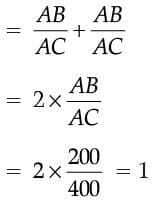Case Based Questions Test: Introduction to Trigonometry - Question 9

Read the following text and answer the following question on the basis of the same:

'Skysails' is that genre of engineering science that uses extensive utilization of wind energy to move a vessel in the sea water. The sky sails technology allows the towing kite to gain a height of anything between 100 m to 300 m. The sailing kite is made in such a way that it can be raised to its proper elevation and then brought back with the help of a telescopic mast that enables the kite to be raised properly and effectively.Q. In the given figure, if tan θ = cot (30° + θ), where θ and 30° + θ are acute angles, then the value of θ is:

Detailed Solution for Case Based Questions Test: Introduction to Trigonometry - Question 9 Given, tan θ = cot(30° + θ)

= tan[90° – (30° + θ)]

= tan(90° – 30° – θ)

⇒ tan θ = tan(60° – θ)

⇒ θ = 60° – θ

⇒ 2θ = 60°

⇒ θ = 30°.

Case Based Questions Test: Introduction to Trigonometry - Question 10

Read the following text and answer the following question on the basis of the same:

'Skysails' is that genre of engineering science that uses extensive utilization of wind energy to move a vessel in the sea water. The sky sails technology allows the towing kite to gain a height of anything between 100 m to 300 m. The sailing kite is made in such a way that it can be raised to its proper elevation and then brought back with the help of a telescopic mast that enables the kite to be raised properly and effectively.Q. What should be the length of the rope of the kite sail in order to pull the ship at the angle θ and be at a vertical height of 200 m?

Detailed Solution for Case Based Questions Test: Introduction to Trigonometry - Question 10 In ΔABC, we have

θ = 30°, AB = 200 m

Then, sin 30° = Perpendicular / Hypotenuse

= AB / AC

⇒ 1 / 2 = 200 / AC

⇒ AC = 400 m.

 Use Code STAYHOME200 and get INR 200 additional OFF Use Coupon Code
Information about Case Based Questions Test: Introduction to Trigonometry Page
In this test you can find the Exam questions for Case Based Questions Test: Introduction to Trigonometry solved & explained in the simplest way possible. Besides giving Questions and answers for Case Based Questions Test: Introduction to Trigonometry, EduRev gives you an ample number of Online tests for practice